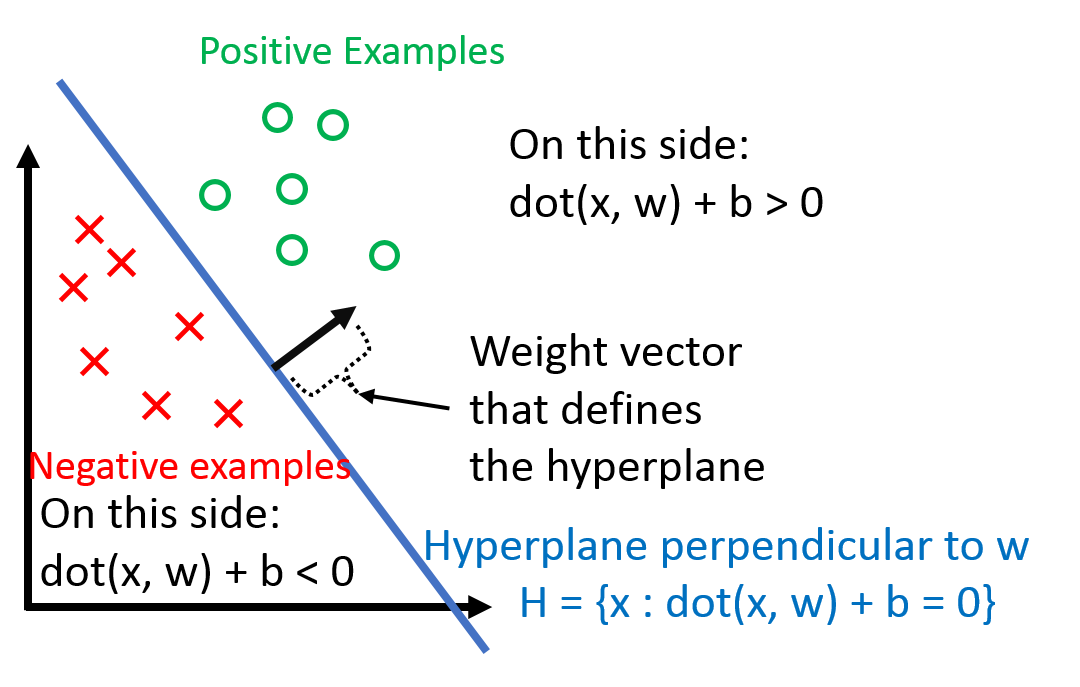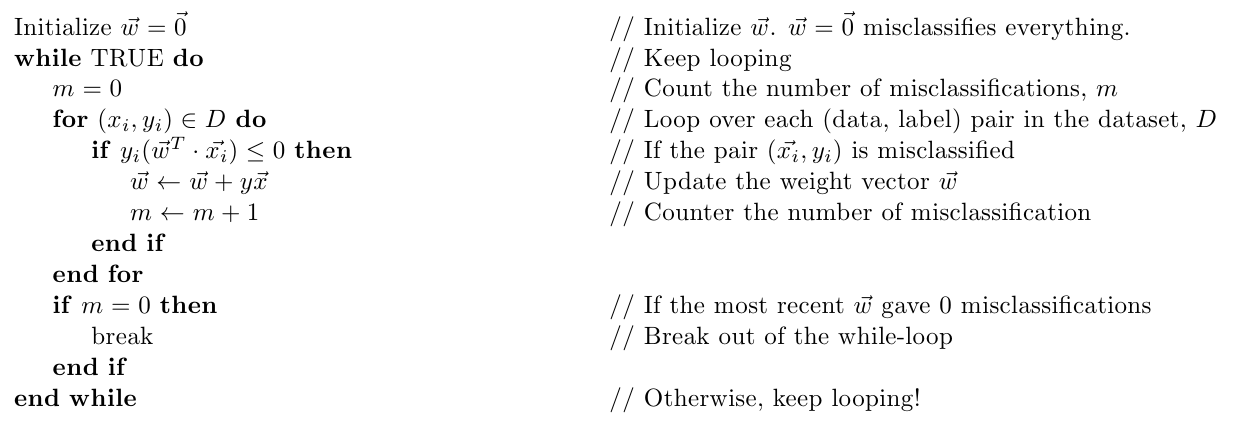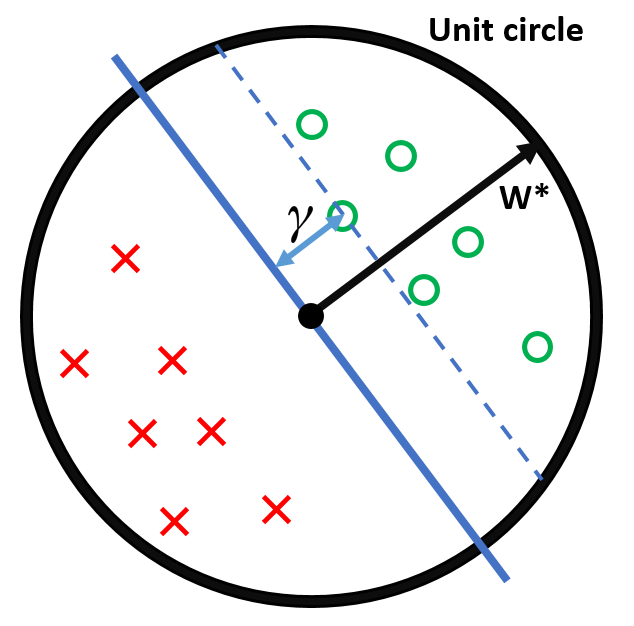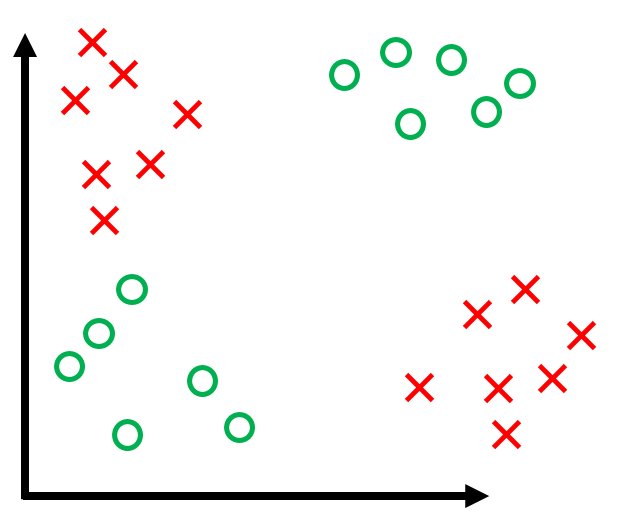## Lecture 3: The Perceptron

### Assumptions

1. Binary classification (i.e. $y_i \in \{-1, +1\}$)
2. Data is linearly separable

### Classifier

$$h(x_i) = \textrm{sign}(\vec{w} \cdot \vec{x_i} + b)$$$b$ is the bias term (without the bias term, the hyperplane that $\vec{w}$ defines would always have to go through the origin). Dealing with $b$ can be a pain, so we 'absorb' it into the feature vector $\vec{w}$ by adding one additional constant dimension. Under this convention, $$\vec{x_i} \hspace{0.1in} \text{becomes} \hspace{0.1in} \begin{bmatrix} \vec{x_i} \\ 1 \end{bmatrix} \\ \vec{w} \hspace{0.1in} \text{becomes} \hspace{0.1in} \begin{bmatrix} \vec{w} \\ b \end{bmatrix} \\$$ We can verify that $$\begin{bmatrix} \vec{x_i} \\ 1 \end{bmatrix} \cdot \begin{bmatrix} \vec{w} \\ b \end{bmatrix} = \vec{w} \cdot \vec{x_i} + b$$ Using this, we can simplify the above formulation of $h(x_i)$ to $$h(x_i) = \textrm{sign}(\vec{w} \cdot \vec{x})$$ Observation: Note that $$y_i(\vec{w} \cdot \vec{x_i}) > 0 \Longleftrightarrow x_i \hspace{0.1in} \text{is classified correctly}$$ where 'classified correctly' means that $x_i$ is on the correct side of the hyperplane defined by $\vec{w}$. Also, note that the left side depends on $y_i \in \{-1, +1\}$ (it wouldn't work if, for example $y_i \in \{0, +1\}$).

### Perceptron Algorithm

Now that we know what the $\vec{w}$ is supposed to do (defining a hyperplane the separates the data), let's look at how we can get such $\vec{w}$.

Perceptron Algorithm#### Geometric Intuition

Quiz#1: Can you draw a visualization of a Perceptron update?
Quiz#2: How often can a Perceptron misclassify a point $\vec{x}$ repeatedly?

### Perceptron Convergence

Suppose that $\exists \vec{w}^*$ such that $y_i(\vec{w}^* \cdot \vec{x}) > 0$ $\forall (\vec{x}_i, y_i) \in D$.

Now, suppose that we rescale each data point and the $\vec{w}^*$ such that $$||\vec{w}^*|| = 1 \hspace{0.3in} \text{and} \hspace{0.3in} ||\vec{x_i}|| \le 1 \hspace{0.1in} \forall \vec{x_i} \in D$$ The Margin of a hyperplane, $\gamma$, is defined as $$\gamma = \min_{(\vec{x_i}, y_i) \in D}|\vec{w}^* \cdot \vec{x_i} |$$ We can visualize this as follows• All inputs $\vec{x_i}$ live within the unit sphere
• $\gamma$ is the distance from the hyperplane (blue) to the closest data point
• $\vec{w}^*$ lies on the unit sphere

Theorem: If all of the above holds, then the perceptron algorithm makes at most $1 / \gamma^2$ mistakes.

Proof:
Keeping what we defined above, consider the effect of an update ($\vec{w}$ becomes $\vec{w}+y\vec{x}$) on the two terms $\vec{w} \cdot \vec{w}^*$ and $\vec{w} \cdot \vec{w}$. We will use two facts:
• $y( \vec{x}\cdot \vec{w})\leq 0$: This holds because $\vec x$ is misclassified by $\vec{w}$ - otherwise we wouldn't make the update.
• $y( \vec{x}\cdot \vec{w}^*)>0$: This holds because $\vec{w}^*$ is a separating hyper-plane and classifies all points correctly.
1. Consider the effect of an update on $\vec{w} \cdot \vec{w}^*$: $$(\vec{w} + y\vec{x}) \cdot \vec{w}^* = \vec{w} \cdot \vec{w}^* + y(\vec{x}\cdot \vec{w}^*) \ge \vec{w} \cdot \vec{w}^* + \gamma$$ The inequality follows from the fact that, for $\vec{w}^*$, the distance from the hyperplane defined by $\vec{w}^*$ to $\vec{x}$ must be at least $\gamma$ (i.e. $y (\vec{x}\cdot \vec{w}^*)=|\vec{x}\cdot\vec{w}^*|\geq \gamma$).

This means that for each update, $\vec{w} \cdot \vec{w}^*$ grows by at least $\gamma$.
2. Consider the effect of an update on $\vec{w} \cdot \vec{w}$: $$(\vec{w} + y\vec{x})\cdot (\vec{w} + y\vec{x}) = \vec{w} \cdot \vec{w} + 2y(\vec{w} \cdot\vec{x}) + y^2(\vec{x}\cdot \vec{x}) \le \vec{w} \cdot \vec{w} + 1$$ The inequality follows from the fact that
• $2y(\vec{w}\cdot \vec{x}) < 0$ as we had to make an update, meaning $\vec{x}$ was misclassified
• $y^2(\vec{x} \cdot \vec{x}) \le 1$ as $y^2 = 1$ and all $\vec{x}\cdot \vec{x}\leq 1$ (because $\|\vec x\|\leq 1$).
3. This means that for each update, $\vec{w} \cdot \vec{w}$ grows by at most 1.
4. Now we can put together the above findings. Suppose we had $M$ updates. \begin{align} M\gamma &\le \vec{w}\cdot\vec{w}^* &&\text{By (1)} \\ &= |\vec{w}\cdot\vec{w}^*| &&\text{By (1) again (the dot-product must be non-negative because the initialization is 0 and each update increases it by at least $\gamma$)} \\ &\le ||\vec{w}||\ ||\vec{w}^*|| &&\text{By Cauchy-Schwartz inequality} \\ &= ||\vec{w}|| &&\text{As $||\vec{w}^*|| = 1$} \\ &= \sqrt{\vec{w} \cdot \vec{w}} && \text{by definition of $\|\vec{w}\|$} \\ &\le \sqrt{M} &&\text{By (2)} \\ &\Rightarrow M\gamma \le \sqrt{M} \\ &\Rightarrow M^2\gamma^2 \le M \\ &\Rightarrow M \le \frac{1}{\gamma^2} \end{align}

### History

• Initially, huge wave of excitement ("Digital brains")
• Then, contributed to the A.I. Winter. Famous counter-example XOR problem (Minsky 1969):
•• If data is not linearly separable, it loops forver.